# Inverse Trigonometric Functions in Maths

Trigonometry is a measurement of triangle and it is included with inverse functions. sin-1x, cos-1 x, tan-1 x etc. represent angles or real numbers and their sine is x, cosine is x and tangent is x , given that the answers are numerically smallest available. These are also written as arc sin x, arc cos x etc.

If there are two angles, one positive and another negative having the same numerical value, then positive angle should be taken. In this article, we will illustrate about the topic of “inverse trigonometric functions” , important formulas and some solved problems.

## Principal Values and Domain of Inverse Trigonometric Functions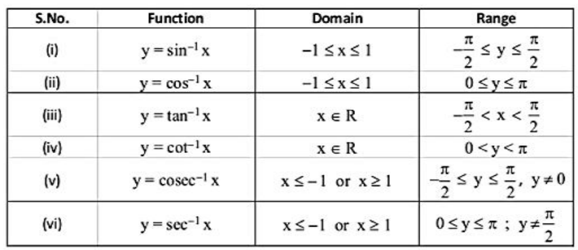## Formulas of Inverse Trigonometric Functions

Given below are some basic formulas for inverse of trigonometric functions:

$\sin^{-1} (\frac{1}{x}) = \csc ^{-1} x$ $\cos ^{-1} (\frac{1}{x}) = \sec ^{-1} x$ $\tan ^{-1} (\frac{1}{x}) = \cot ^{-1} x$ $\sin ^{-1} (-x) = – \sin ^{-1} x$ $\tan ^{-1} x + \cot ^{-1} x = \frac{\pi}{2}$ $\sin ^{-1} x + \cos ^{-1} x = \frac{\pi}{2}$ $\csc ^{-1} x + \sec ^{-1} x = \frac{\pi}{2}$ $\tan ^{-1} x + \tan ^{-1} y = \tan ^{-1} (\frac{x + y}{1 – xy})$ $\tan ^{-1} x – \tan ^{-1} y = \tan ^{-1} (\frac{x + y}{1 – xy})$ $2 \tan ^{-1} x = \sin ^{-1} (\frac{2x}{1 + x^2}) = \cos ^{-1} (\frac{1 – x^2}{1 + x^2}) = \tan ^{-1}(\frac{2x}{1 – x^2})$

## Graphs of all Six Inverse Circular Functions

(1) Arc Sin x

Let y = sin^(-1) x, |x| ≤1, y ∈ [(-Π)/2, Π/2]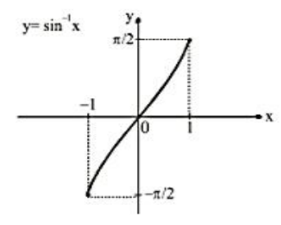Important Points: (i) sin-1 x is bounded in [(-Π)/2, Π/2] (ii) sin-1 x is an odd function. (symmetric about origin) (iii) sin-1 x is an increasing function in its domain. (iv) Maximum value of sin-1 x = Π/2, occurs at x = 1 and minimum value of sin-1 x = (-Π)/2, occurs at x = -1 (v) sin-1 x is a periodic function.

(2) Arc Cos x

Let y = cos-1 x, |x| ≤ 1, y ∈[0 , Π ]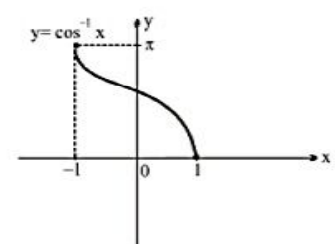Important Points: (i) cos-1 x is bounded in [0 ,Π ] (ii) cos-1 x is a neither odd nor even function. (iii) cos-1 x is a decreasing function in its domain. (iv) Maximum value of cos-1 x= Π, occurs at x=-1 and minimum value of cos-1 x= 0, occurs at x=1. (v) cos-1x is a periodic function.

(3) Arc tan x

Let y = tan-1  x, x ϵ R , y ∈ ((-Π)/2, Π/2)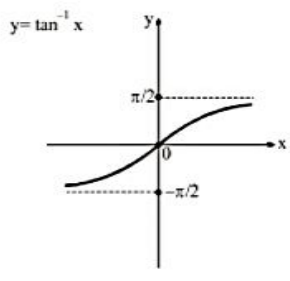Important Points: (i) tan-1 x is bounded in ((-Π)/2,Π/2) (ii) tan-1  x is an odd function(symmetric about x axis). (iii) tan-1 ) x is an increasing function in its domain. (iv) Maximum and minimum value is not defined for the tan-1  x. (v) tan-1 x is a periodic function.

(4) Arc cot x

Let y = cot-1  x, x ϵ R, y ∈(0 , Π )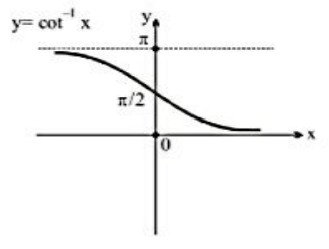Important points: (i) cot-1  x is bounded in (0 , Π ) (ii) cot-1 ) x is a neither odd nor even function. (iii) cot-1 ) x is a decreasing function in its domain. (iv) Maximum and minimum value is not defined for the cot-1  x. (v) cot-1  x is a periodic function.

(5) Arc Sec x

Let y = sec-1  x, |x| ≥ 1 , y ∈ [0, Π/2) ∪ (Π/2, Π]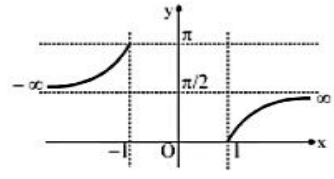Important points: (i) sec-1  x is bounded in [0 ,Π] (ii) sec-1  x is a neither odd nor even function. (iii) sec-1  x is an increasing function in two different intervals. (iv) Maximum value of sec-1  x is π, occurs at x = -1 and minimum value of the sec-1  x is -π, occurs at x = 1. (v) sec-1  x is a periodic function.

(6) Arc cosec x

Let y = cosec^(-1) x, |x|≥1 , y ∈[-Π/2,0) ∪ (0,Π/2]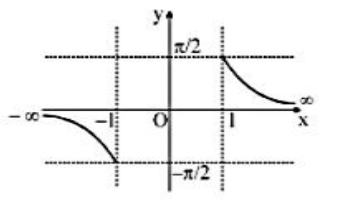Important points: (i) cosec-1x is bounded in [-Π/2,Π/2] (ii) cosec-1 x is an odd function(symmetric about origin). (iii) cosec-1 x is a decreasing function in two different intervals. (iv) Maximum value of cosec-1 x is Π/2, occurs at x = 1 and minimum value of the cosec-1 x is -Π/2, occurs at x = -1. (v) cosec-1 x is a periodic function.

## Solved Examples

Example 1: Find the value of sin(2 sin-1 3/5).

Solution: let sin-1 3/5= A

⇒ sin A = 3/5, cos A = 4/5

⇒ sin (2sin-1 3/5) = sin 2A = 2.sin A.cos A = 2.3/5.4/5=24/25.

Example 2: Find the value of cos(sin-14/5 – cos-1 4/5)

Solution: Let sin-1 4/5 = A and cos-1 4/5 = B

⇒ sin A = 4/5 and cos B = 4/5

⇒ cos A = 3/5 and sin B = 3/5

⇒ cos(sin-1 4/5 – cos-1 4/5)

[Using cos(A-B) = cos A cos B + sin A sin B]

⇒ 4/5 x 3/5 + 4/5 x 3/5 = 24/25.

Example 3: Show that $sin^{-1}(2x \sqrt{1 – x^2}) = 2 cos ^{-1}(x), \frac{-1}{\sqrt{2}} \leq x \leq 1$

Solution:

Given $sin^{-1}(2x \sqrt{1 – x^2}) = 2 cos ^{-1}(x)$

Let us consider $x = \cos \theta$

Then, $cos^{-1} x = \theta$

We have

$sin^{-1}(2x \sqrt{1 – x^2}) = sin^{-1}(2 cos \theta \sqrt{1 – \cos^2 \theta})$

Formula: $sin^2 \theta = 1 – cos^2 \theta$

= $sin^{-1} (2 cos \theta \sqrt{sin ^2 \theta})$

= $sin^{-1}(2 cos \theta sin \theta)$

Formula: $sin 2 \theta = 2 sin \theta cos \theta$

= $sin^{-1}(sin 2 \theta)$

= $2 \theta$

= $2 cos ^{-1} (x)$

Example 4: Write $tan^{-1} (\frac{cos y}{1 – sin y}), \frac{-\pi}{2} < y < \frac{\pi}{2}$ in the simplest form.

Solution:

Given, $tan^{-1} (\frac{cos y}{1 – sin y})$

We are going to simplify the given $tan^{-1} \frac{cos y}{1 – sin y}$

Formula: $cos y = sin (\frac{\pi}{2} – y)$ and $sin y = cos (\frac{\pi}{2} – y)$ $tan^{-1}( \frac{cos y}{1 – sin y}) = tan^{-1}\frac{sin(\frac{\pi}{2} – y)}{1 – cos (\frac{\pi}{2} – y)}$

= $tan ^{-1} (tan (\frac{\pi}{4} + \frac{y}{2})$)

= $\frac{\pi}{4} + \frac{y}{2}$